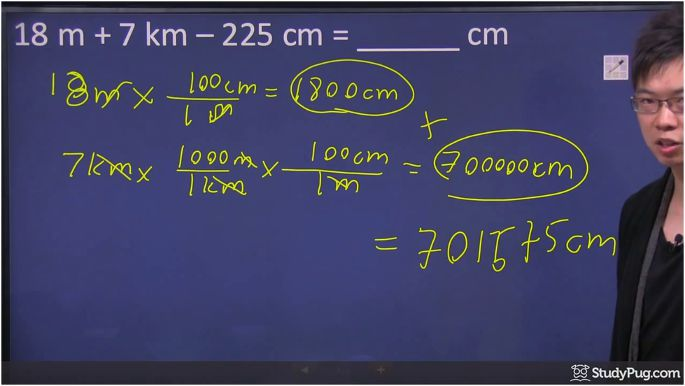# Metric systems

## metric conversion

Metric system, as known as International System of Units, is an internationally recognized system of measurement. Metric units are multiples of ten of the base unit. The three most common units in the metric system are the meter, gram and liter. The prefixes of these units are: kilo-, hecto-, deka-, centi-, and milli-. In order to better help you remember the order of the units' sizes, use this memory aid:

King Henry Doesn't (Usually) Drink Chocolate Milk

The first letter of each of the above words correspond to the different prefixes, with the "U" in "Usually" standing for "unit", which reminds you that there's a unit of either meters, grams, or liters. Each of these prefixes are 10 times or 1/10th of the units on either side of them. So for example:

1 kilogram = 10 hectagram = 100 dekagram = (1000 grams) = 10,000 decigrams = 100,000 centigrams = 1,000,000 milligrams

As you can see, carrying out metric conversion between units is relatively simple as they're all multiples of tens of one another. This means that by moving the decimal point, you'll be able to convert between them. For a general rule, when you move from a bigger unit to a smaller unit (a unit that's to the right in the above memory aid), move the decimal place to the right an equal number of places. The same is said for moving left, when we move from a smaller number to a bigger one in unit conversion.

In this tutorial we will show you how to convert cm to m (centimeter to meter) and m to km (meter to kilometer). Refer to the below metric conversion table to help guide you.

How do we do questions with multiple different units and how do we do unit conversion, then combine them into a single unit?

Let's take this question for example. Here, we've got 18 meters plus 7 kilometers and another 225 centimeters. You are trying to convert everything into centimeters.

The question will involve converting meter to cm, kilometer to m, as well as some addition.

We can add or subtract these numbers if they're all in the same unit, so we are going to change everything to centimeters because the question is looking for an answer in centimeters. Always check which unit in the metric system you're supposed to be calculating for.

## m to cm

Here we've got 18 meters in this question. Since we are trying to change from meters to centimeters, how many centimeters in a meter? From the metric conversion table we provided above, we know there are 100 centimeters per every meter. All you have to do is to multiply 18m by 100cm. The meter gets cancelled out and we are left with 1800 centimeters.

## km to cm

The next term is 7 kilometer.

Let's go from kilometers to meters first because that's easier. We first must ask: how many meters in a kilometer?

For every thousand meters, it's equivalent to one kilometer. We also know that every 100 centimeters is equivalent to 1 meter.

So we have 7km times 1000m times 100cm.

In the end, when you have kilometer cancelled out, as well as your meters cancelled out, you're again left with the centimeter, which is 700,000 centimeters.

If you add together all the centimeters we've got from the above steps, you should get 701,800.

Take the 700,000cm and add the 1800cm we've got from our previous step. Then, subtract the 225cm from the question.

The final answer comes to 701,575cm. You've successfully carried out unit conversion and used it to solve the problem by converting from km to m, then to cm.everything converted into centimeters, and that's the final answer

If you need to double check your work, feel free to reference this online metric converter, or this one that includes a wider variety of different measurements.

### Metric systems

Metric system, as known as International System of Units, is an internationally recognized system of measurement. Metric units are multiples of ten of the base unit. The three most common units in the metric system are the meter, gram and liter.

#### Lessons

$\cdot$ SI = International Systems of Units (official systems in Canada)
$\cdot$Based on number of 10

Lengths
1 km = 1000 m
or
1 m = 0.001 km
1 m = 100 cm
or
1 cm = 0.01 m
1 cm = 10 mm
or
1 mm = 0.1 cm
• Introduction
a)
What is the Metric system?

• 1.
Completing the conversions
a)
0.4 km =____ m

b)
45 mm =____ km

c)
54 m =____ mm

• 2.
Add or subtract different metric units
a)
18 m + 7 km - 225 cm = ______ cm

b)
1500 cm + 20 m - 0.03 km = ______ m

c)
0.35km + 5000 cm - 200 m = _____ mm